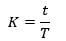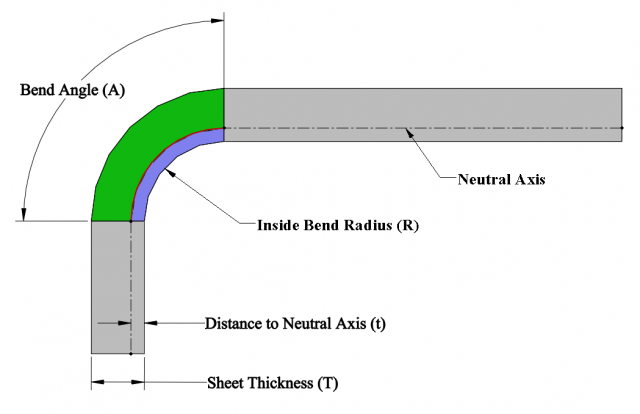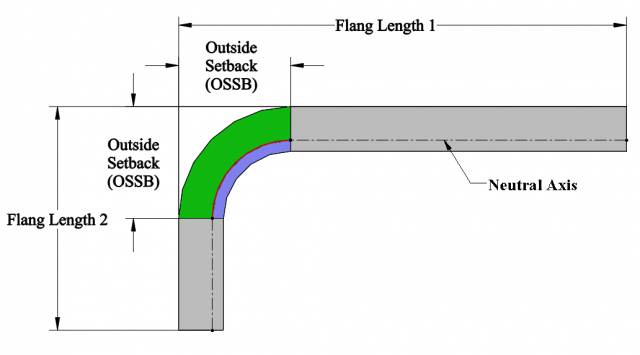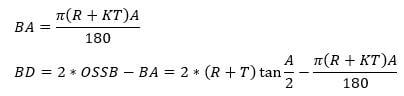# What Are Bend Allowance, Bend Deduction and K-Factor?Article by Saeed Mojarad (CSWE) updated June 1, 2017

###### Article

In a series of posts we are going to review the most important sheet metal design terminology — Bend Allowance, Bend Deduction and K-Factor. In this post I will review what these terms mean and in the next post I will show you how to calculate and use them in SOLIDWORKS.

In sheet metal bending process when metal is bent the material along the inner bend radius is going to undergo compression and the material along the outer bend radius will be stretched. The line where the transition from compression to stretching occurs is called the neutral axis or the neutral sheet. The neutral axis is where neither the material stretches nor compressed. Hence, the length of the neutral axis stay the same before and after bending operation. The location of the neutral axis varies and is based on the material’s physical properties and its thickness. It is important to know the location of the neutral axis for a specific sheet as we do all our calculations based on the neutral axis to calculate flat patterns. The location of the neutral axis for a specific sheet is defined by a factor called K-Factor.

### K-Factor

K-Factor is a ratio that represents the location of the neutral axis with respect to the thickness of the sheet metal part and depends on material, thickness and bend radius. K-Factor (K) can be defined as follows:Where t is the distance from inside face to the neutral sheet and T is the sheet thickness (Figure 1). In practice, K-Factor is used when you don’t know the process or machine that is going to be used to bend the sheet.Figure 1: Neutral axis of a bended sheet

### Bend Allowance

The Bend Allowance (BA) is the arc length of the bend as measured along the neutral axis of the material. Understanding the Bend Allowance and consequently the Bend Deduction of a part is a crucial first step to understanding how sheet metal parts are fabricated.

When the sheet metal is put through the process of bending the metal around the bend is deformed and stretched. As this happens you gain a small amount of total length in your part. The Bend Allowance is defined as the material you will have to add to the initial length of your flat sheet in order to arrive at the length of formed part. As it was mentioned before the length of the neutral axis doesn’t change after the bending. So the following equation is valid at all times:

`Initial Length = Leg Length 1 + BA + Leg Length 2`Figure 2: Bend Allowance

# Need Help with your Sheet Metal Setup?

Our SOLIDWORKS Experts can setup your environment so that your team uses a comprehensive set of templates, tables, and library of forming tools

### Bend Deduction

On the other hand, when you are trying to develop a flat pattern you will have to make a deduction from your desired part size to get the correct flat size. The Bend Deduction is defined as the material you will have to remove from the total length of your flanges in order to arrive at the flat pattern. To calculate the Ben Deduction, we can rewrite the previous equation as:

```Initial Length = Leg Length 1 + BA + Leg Length 2

Initial Length = ( Flang Length 1 - OSSB)  + BA + ( Flang Length 2 - OSSB)

Initial Length = Flang Length 1  + Flang Length 2 - (2*OSSB - BA)```

The last term of the previous equation is called the Bend Deduction which is the difference between the Bend Allowance and twice the outside setback.

`Bend Deduction = 2*OSSB - BA`Figure 3: Outside Setback (OSSB)

### Calculating Bend Allowance and Bend Deduction using K-Factor

Bend Allowance and Bend Deduction can be calculated using the K-Factor as follows:If you are interested in how to measure the Bend Allowance, Bend Deduction and K-Factor for different situations you can read my next blog post.

#### Get Certified SOLIDWORKS Services from Javelin